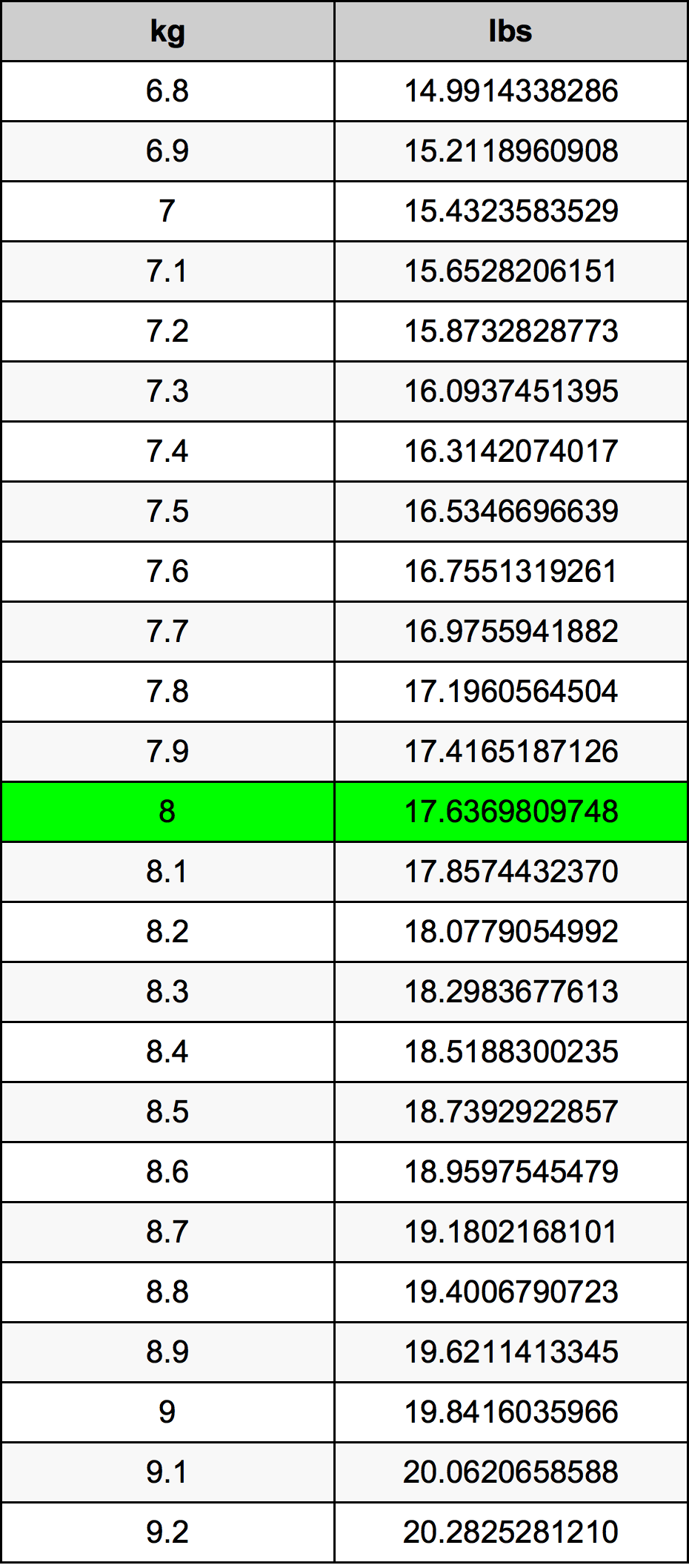Kg To Lbs

8 kg to lbs8 Kilograms to Pounds

kg
=
lbs

How to convert 8 kilograms to pounds?

 8 kg * 2.2046226218 lbs = 17.6369809748 lbs 1 kg
A common question is How many kilogram in 8 pound? And the answer is 3.62873896 kg in 8 lbs. Likewise the question how many pound in 8 kilogram has the answer of 17.6369809748 lbs in 8 kg.

How much are 8 kilograms in pounds?

8 kilograms equal 17.6369809748 pounds (8kg = 17.6369809748lbs). Converting 8 kg to lb is easy. Simply use our calculator above, or apply the formula to change the length 8 kg to lbs.

Convert 8 kg to common mass

UnitMass
Microgram8000000000.0 µg
Milligram8000000.0 mg
Gram8000.0 g
Ounce282.191695597 oz
Pound17.6369809748 lbs
Kilogram8.0 kg
Stone1.2597843553 st
US ton0.0088184905 ton
Tonne0.008 t
Imperial ton0.0078736522 Long tons

What is 8 kilograms in lbs?

To convert 8 kg to lbs multiply the mass in kilograms by 2.2046226218. The 8 kg in lbs formula is [lb] = 8 * 2.2046226218. Thus, for 8 kilograms in pound we get 17.6369809748 lbs.

8 Kilogram Conversion TableAlternative spelling

8 kg to lbs, 8 kg in lbs, 8 Kilogram to lb, 8 Kilogram in lb, 8 Kilogram to Pound, 8 Kilogram in Pound, 8 Kilogram to lbs, 8 Kilogram in lbs, 8 Kilograms to Pounds, 8 Kilograms in Pounds, 8 kg to Pound, 8 kg in Pound, 8 Kilograms to Pound, 8 Kilograms in Pound, 8 kg to Pounds, 8 kg in Pounds, 8 Kilograms to lb, 8 Kilograms in lb